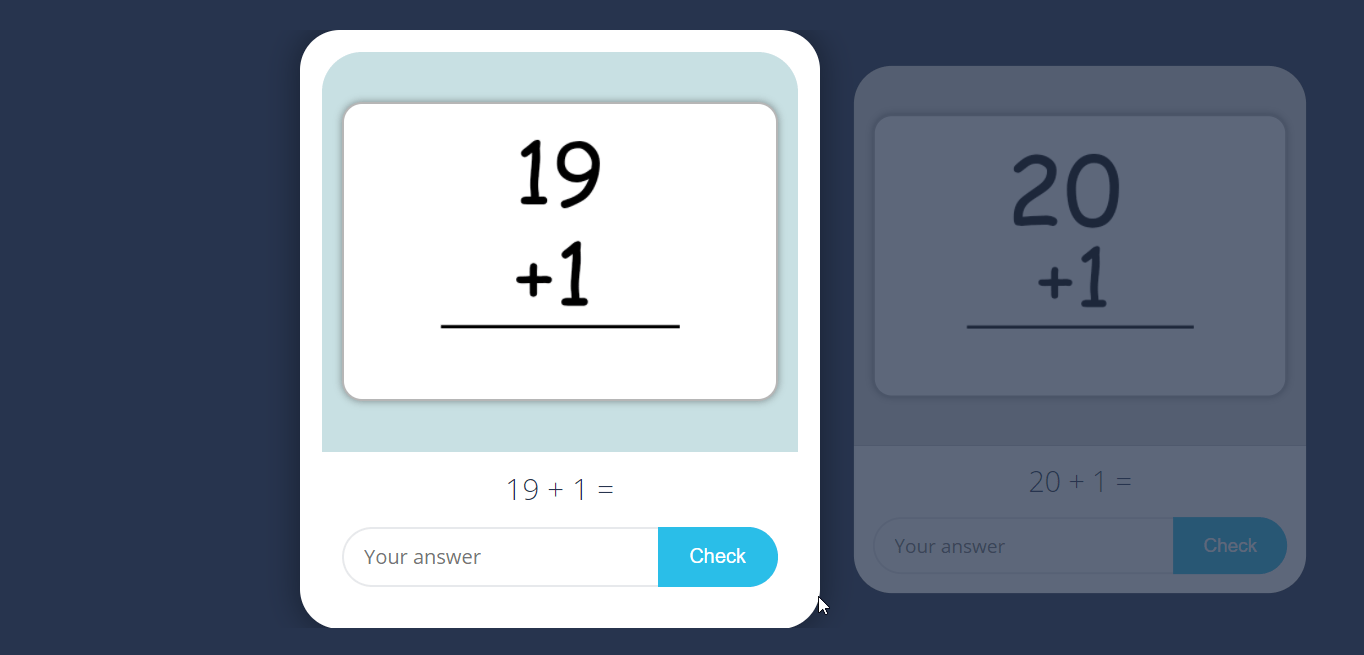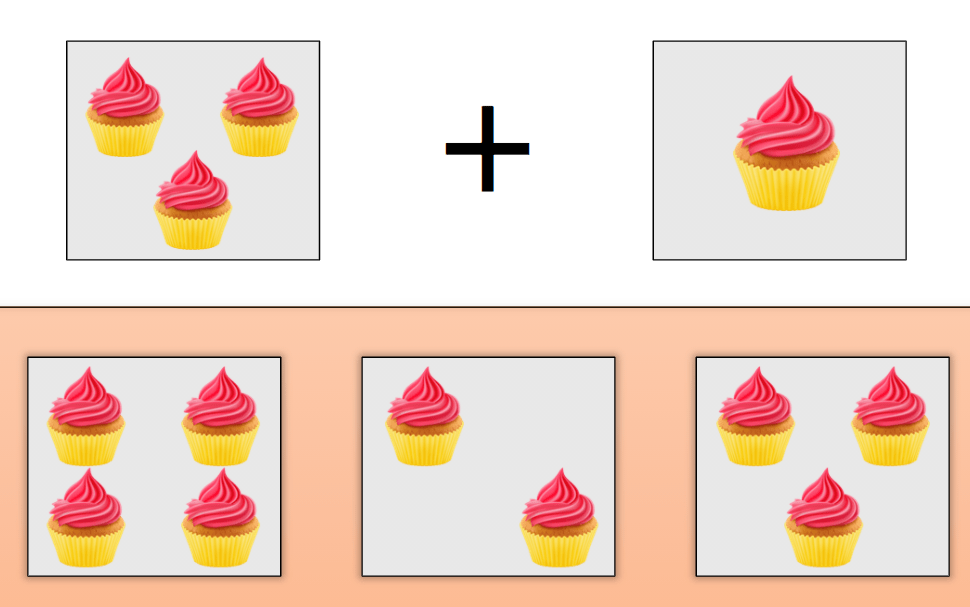# Maths Level 1This online Maths course about addition without carry over has various interactive activities and educational games. These activities make the concept of addition easy, simple and fun. Addition sums without carry over are a useful steeping stone for learning more complex addition sums. All the addition sums without carry over in this course are such …## Subtraction upto 5

In this subtraction course, students will learn the concept of subtraction. The subtraction sums or problems will be limited upto subtraction from 5. These interactive subtraction worksheets or educational games will provide a simple method of learning about subtraction. With picture based subtraction, subtraction on a number line, some simple subtraction rules and some word …